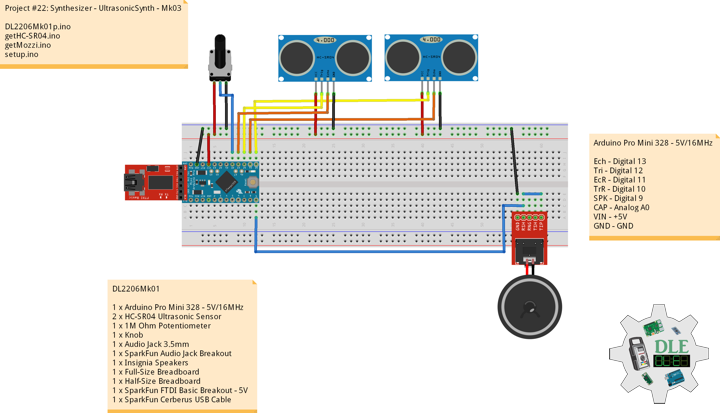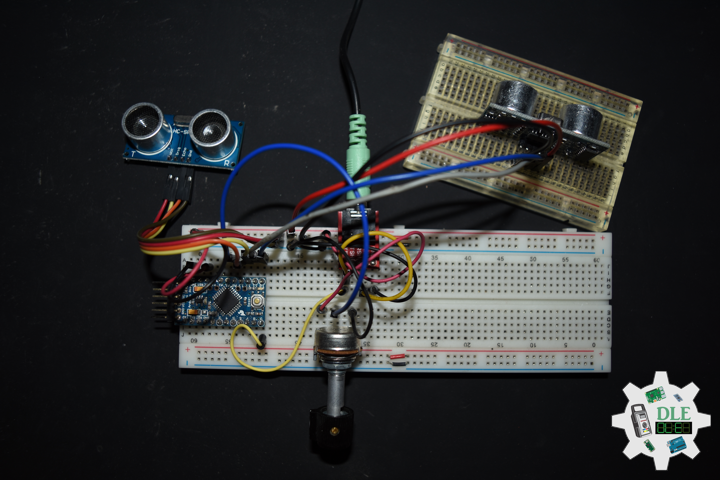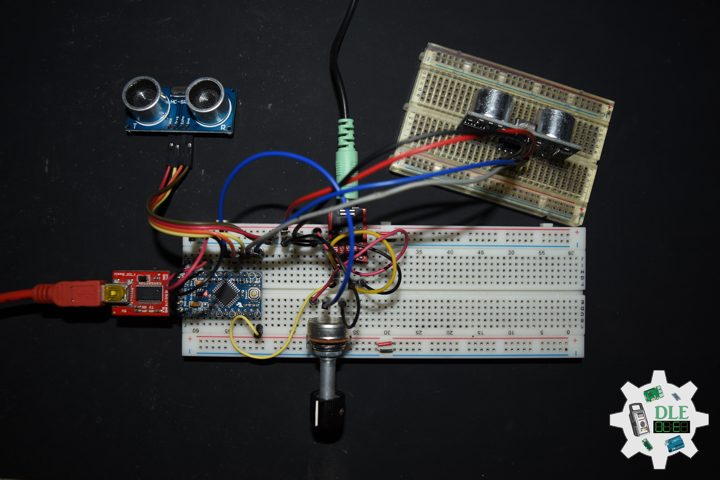# Project #22: Synthesizer – UltrasonicSynth – Mk03

——

#DonLucElectronics #DonLuc #Synthesizer #UltrasonicSynth #Arduino #ArduinoProMini #Project #Fritzing #Programming #Electronics #Microcontrollers #Consultant

————————

UltrasonicSynth Mozzi

Oscil

Oscil plays a wavetable, cycling through the table to generate an audio or control signal. The frequency of the signal can be set or changed, and the output of an Oscil can be produced with for a simple cycling oscillator, or for a particular sample in the table.

Soundtables

Look-up-tables and python scripts to generate tables or convert sounds. Includes ready-to-use wave tables and a few example samples. Also check out the other scripts in the python folder for templates to use if you want to do your own thing.

Smooth

A simple infinite impulse response low pass filter for smoothing control or audio signals. Smoothness sets how much smoothing the filter will apply to its input. Use a float in the range 0 – 1, where 0 is not very smooth and 0.99 is very smooth.

AutoMap

Automatically map an input value to an output range without knowing the precise range of inputs beforehand.

DL2204Mk01

1 x Arduino Pro Mini 328 – 5V/16MHz
2 x HC-SR04 Ultrasonic Sensor
1 x 1M Ohm Potentiometer
1 x Knob
1 x Audio Jack 3.5mm
1 x SparkFun Audio Jack Breakout
1 x Insignia Speakers
1 x SparkFun FTDI Basic Breakout – 5V
1 x SparkFun Cerberus USB Cable

Arduino Pro Mini 328 – 5V/16MHz

Ech – Digital 13
Tri – Digital 12
EcR – Digital 11
TrR – Digital 10
SPK – Digital 9
CAP – Analog A0
VIN – +5V
GND – GND

——

DL2206Mk01p.ino

```/* ***** Don Luc Electronics © *****
Software Version Information
Project #22: Synthesizer - UltrasonicSynth - Mk03
22-03
DL2206Mk01p.ino
1 x Arduino Pro Mini 328 - 5V/16MHz
2 x HC-SR04 Ultrasonic Sensor
1 x 1M Ohm Potentiometer
1 x Knob
1 x Audio Jack 3.5mm
1 x SparkFun Audio Jack Breakout
1 x Insignia Speakers
1 x SparkFun FTDI Basic Breakout - 5V
1 x SparkFun Cerberus USB Cable
*/

// Include the Library Code
// Mozzi
#include <MozziGuts.h>
// Oscillator
#include <Oscil.h>
// Table for Oscils to play
#include <tables/cos2048_int8.h>
// Smoothing Control
#include <Smooth.h>
// Maps unpredictable inputs to a range
#include <AutoMap.h>

// Desired carrier frequency max and min, for AutoMap
const int MIN_CARRIER_FREQ = 22;
const int MAX_CARRIER_FREQ = 440;

// Desired intensity max and min, for AutoMap, note they're inverted for reverse dynamics
const int MIN_INTENSITY = 450;
const int MAX_INTENSITY = 50;

// Desired mod speed max and min, for AutoMap, note they're inverted for reverse dynamics
const int MIN_MOD_SPEED = 450;
const int MAX_MOD_SPEED = 50;

// Maps unpredictable inputs to a range
AutoMap kMapCarrierFreq(0,1023,MIN_CARRIER_FREQ,MAX_CARRIER_FREQ);
AutoMap kMapIntensity(0,1023,MIN_INTENSITY,MAX_INTENSITY);
AutoMap kMapModSpeed(0,1023,MIN_MOD_SPEED,MAX_MOD_SPEED);

// Set the input for the knob to analog pin 0
const int KNOB_PIN = A0;
int LDR1_PIN;
int LDR2_PIN;

// Table for Oscils to play
Oscil<COS2048_NUM_CELLS, AUDIO_RATE> aCarrier(COS2048_DATA);
Oscil<COS2048_NUM_CELLS, AUDIO_RATE> aModulator(COS2048_DATA);
Oscil<COS2048_NUM_CELLS, CONTROL_RATE> kIntensityMod(COS2048_DATA);

// Brightness (harmonics)
int mod_ratio = 5;
// Carries control info from updateControl to updateAudio
long fm_intensity;

// Smoothing for intensity to remove clicks on transitions
float smoothness = 0.95f;
Smooth <long> aSmoothIntensity(smoothness);

// Trigger pin 12 to pitch distance sensor
const int iTrigPitch = 12;
// Echo Receive pin 13 to pitch distance sensor
const int iEchoPitch = 13;
// Define the useable range of the pitch sensor
const int pitchLowThreshold = 450;
const int pitchHighThreshold = 50;
// Stores the distance measured by the distance sensor
float distance = 0;
// Trigger pin 10 to rate distance sensor
const int iTrigRate = 10;
// Echo Receive pin 13 to pitch distance sensor
const int iEchoRate = 11;
// Define the useable range of the pitch sensor
const int rateLowThreshold = 450;
const int rateHighThreshold = 50;
// Stores the distance measured by the distance sensor
float rate = 0;

// Mini Speaker
int SPK = 9;

// Software Version Information
String sver = "22-03";

void loop() {

// Audio Hook
audioHook();

}
```

getHC-SR04.ino

```// HC-SR04 Ultrasonic Sensor
// Setup HC-SR04
void setupHCSR04() {

// The trigger iTrig Pitch will output pulses of electricity
pinMode(iTrigPitch, OUTPUT);
// The echo iEcho will measure the duration of pulses coming back from the distance sensor
pinMode(iEchoPitch, INPUT);

// The trigger iTrig Rate will output pulses of electricity
pinMode(iTrigRate, OUTPUT);
// The echo iEcho will measure the duration of pulses coming back from the distance sensor
pinMode(iEchoRate, INPUT);

}
// Distance
float isDistance() {

// Variable to store the time it takes for a ping to bounce off an object
float echoTime;
// Variable to store the distance calculated from the echo time
float calculatedDistance;

// Send out an ultrasonic pulse that's 10ms long
digitalWrite(iTrigPitch, HIGH);
delayMicroseconds(10);
digitalWrite(iTrigPitch, LOW);

// Use the pulseIn command to see how long it takes for the
// pulse to bounce back to the sensor
echoTime = pulseIn(iEchoPitch, HIGH);

// Calculate the distance of the object that reflected the pulse
// (half the bounce time multiplied by the speed of sound)
// cm = 58.0
calculatedDistance = echoTime / 58.0;

// Send back the distance that was calculated
return calculatedDistance;

}
// Rate
float isRate() {

// Variable to store the time it takes for a ping to bounce off an object
float echoTime;
// Variable to store the distance calculated from the echo time
float calculatedDistance;

// Send out an ultrasonic pulse that's 10ms long
digitalWrite(iTrigRate, HIGH);
delayMicroseconds(10);
digitalWrite(iTrigRate, LOW);

// Use the pulseIn command to see how long it takes for the
// pulse to bounce back to the sensor
echoTime = pulseIn(iEchoRate, HIGH);

// Calculate the distance of the object that reflected the pulse
// (half the bounce time multiplied by the speed of sound)
// cm = 58.0
calculatedDistance = echoTime / 58.0;

// Send back the distance that was calculated
return calculatedDistance;

}
```

getMozzi.ino

```// Mozzi
// Update Control
void updateControl(){

// Variable to store the distance measured by the sensor
distance = isDistance();
// Low Threshold
if ( distance >= pitchLowThreshold) {

// pitchLowThreshold
distance = pitchLowThreshold;

}
// High Threshold
if ( distance < pitchHighThreshold){

// pitchHighThreshold
distance = pitchHighThreshold;

}

// Variable to store the distance measured by the sensor
rate = isRate();
// Low Threshold
if ( rate >= rateLowThreshold) {

// rateLowThreshold
rate = rateLowThreshold;

}
// High Threshold
if ( rate < rateHighThreshold){

// rateHighThreshold
rate = rateHighThreshold;

}

// Value is 0-1023

// Map the knob to carrier frequency
int carrier_freq = kMapCarrierFreq(knob_value);

// Calculate the modulation frequency to stay in ratio
int mod_freq = carrier_freq * mod_ratio;

// Set the FM oscillator frequencies
aCarrier.setFreq(carrier_freq);
aModulator.setFreq(mod_freq);

// Read the light dependent resistor on the width
LDR1_PIN = distance;
int LDR1_value = LDR1_PIN;

int LDR1_calibrated = kMapIntensity(LDR1_value);

// Calculate the fm_intensity
// Shift back to range after 8 bit multiply
fm_intensity = ((long)LDR1_calibrated * (kIntensityMod.next()+128))>>8;

// Read the light dependent resistor on the speed
LDR2_PIN = rate;
int LDR2_value= LDR2_PIN;

// Use a float here for low frequencies
float mod_speed = (float)kMapModSpeed(LDR2_value)/1000;

kIntensityMod.setFreq(mod_speed);

}
// Update Audio
int updateAudio()
{

// Update Audio
long modulation = aSmoothIntensity.next(fm_intensity) * aModulator.next();
return aCarrier.phMod(modulation);

}
```

setup.ino

```// Setup
void setup() {

// Setup HC-SR04
setupHCSR04();

// Delay
delay( 200 );

// Mozzi Start
startMozzi();

}
```

——

Technology Experience

• Single-Board Microcontrollers (PIC, Arduino, Raspberry Pi,Espressif, etc…)
• IoT
• Robotics
• Camera and Video Capture Receiver Stationary, Wheel/Tank and Underwater Vehicle
• Unmanned Vehicles Terrestrial and Marine
• Research & Development (R & D)

Instructor and E-Mentor

• IoT
• PIC Microcontrollers
• Arduino
• Raspberry Pi
• Espressif
• Robotics